Published: 25 June 2019

# Load Analysis of pitch bearing considering non-quenching zone

J. X. Gui1
G. B. Wang2
Z. Zhou3
1, 2Hunan Provincial Key Laboratory of Mechanical Equipment Health, Xiangtan, China
3Xemc-Wind Co., Ltd., Xiangtan, China
Corresponding Author:
J. X. Gui
Views 145

#### Abstract

The pitch bearing of the MW-class wind turbine has a weak zone which is not quenched. In the complicated service environment of the wind turbine, the pitch bearing often has breakage accidents in the non-quenching zone. Firstly, this paper takes the pitch bearing as the object and establishes the pitch bearing model with weak zone. Subsequently, the load variation law of the pitch bearing considering non-quenching is analyzed in the four extreme conditions. Finally, the feasibility of the model is proved by comparing the simulation data with the data obtained from the theoretical formula.

## 1. Introduction

The pitch bearing is an important part of the MW-class wind turbine, and the heat treatment process of the raceway surface is usually quenching. However, the trajectory of the heating head on the raceway cannot overlap (because secondary quenching can lead to cracks), a weak zone will be formed in the non- quenching zone. When the product leaves the factory, the position of the weak zone on the workpiece will be marked with the word “$S$” to indicate the specific weak zone position.

In the current literature, the research on the failure mechanism of the weak zone of the wind turbine pitch bearing is still rare, and more concentrated on the analysis of the mechanical properties and bearing capacity of the pitch bearing without considering the weak zone of the bearing. In foreign studies, Zupan and Prebil studied the effects of initial contact angle, tightness, clearance value and the stiffness of the support structure on the bearing capacity of the bearing and the actual contact angle . Aguirrebeitia established a mathematical model for carrying capacity of bearing . Alain Daidié analyzed the distribution of contact forces experienced by rolling elements under different loads . In the domestic research, Li Y. F. gave the suggestions for the selection of the parameters of the large-scale pitch bearing [4, 5]; Wang H. used the finite element method to calculate the friction torque of single and double pitch bearings respectively ; Mao X. N. verified the strength of the pitch bearing under ABAQUS . In these studies, the influence of the weak zone of the bearing was automatically ignored during the mechanical modeling, and the structural strength and load characteristics of the weak zone were not investigated as the influencing factors during the mechanical analysis.

Therefore, this paper will study the load analysis of pitch bearing considering weak zone.

## 2. Empirical formula

The maximum contact load calculated by empirical formula is still the main form of design analysis of pitch bearings for most pitch bearing manufacturers at present .

When the angular contact radial ball bearing or thrust, bearing is subjected to pure axial force:

1
${Q}_{max}=\frac{{F}_{a}}{Z\mathrm{s}\mathrm{i}\mathrm{n}\alpha }.$

When the ball bearing is subjected to pure radial force:

2
${Q}_{max}=\frac{4.37{F}_{r}}{Z}.$

When the two-way thrust ball bearing is subjected to the overturning moment:

3
${Q}_{max}=\frac{4.37M}{{D}_{w}Z}.$

The pitch bearing is generally subjected to the combined action of the above three loads. According to the characteristics of the pitch bearing structure itself, the empirical formula of the maximum contact load of the pitch bearing subjected to the combined action of three loads is:

4
${Q}_{max}=\frac{{F}_{a}}{Z\mathrm{s}\mathrm{i}\mathrm{n}\alpha }+\frac{2{F}_{r}}{Z\mathrm{c}\mathrm{o}\mathrm{s}\alpha }+\frac{4M}{{D}_{w}Z\mathrm{s}\mathrm{i}\mathrm{n}\alpha }.$

In the formula, the ${F}_{a}$ is axial force, the ${F}_{r}$ is radial force and the M is the overturning moment; the ${D}_{w}$ is diameter of the bearing pitch circle; the $Z$ is number of rolling elements; the $\alpha$ is contact angle.

The design parameters of a pitch bearing are shown in the Table 1.

Table 1A pitch bearing design parameter

 Parameter Data Diameter of outer race mounting hole distribution circle ${D}_{1}$ / mm 2000 Nominal bore diameter $d$ / mm 1716 Diameter of rolling element center circle ${D}_{m}$ / mm 1900 Diameter of inner race mounting hole distribution circle ${d}_{1}$ / mm 1800 Nominal outer diameter $D$ / mm 2080 Rolling body diameter ${D}_{w}$ / mm 45 Inner channel radius of curvature ${r}_{i}$ / mm 23.625 Outer channel radius of curvature ${r}_{e}$ / mm 23.625 Ditch row spacing ${D}_{c}$ / mm 80 Number of mounting holes $N$ 54 Number of rolling elements $Z$ 2×120 Initial contact angle $\alpha$ / (°) 45

## 3. Calculation of bearing weak zone by finite element

### 3.1. Establishment of weak zone model

The hardness of the pitch bearing weak zone is generally around 35 HRC, which is lower than the hardness range of 55-62 HRC in the quenching zone, thus it becomes the weakest zone in the pitch bearing. Looking over the hardness and tensile strength table, the common material elastic modulus, Poisson’s ratio comparison table can get the data in the Table 2.

Table 2Material properties of various parts of pitch bearings

 Part Elastic modulus $E$ (Pa) Poisson’s ratio $\mu$ tensile strength (MPa) Quenching zone 2.12e+011 0.3 2180 Weak zone 2.00e+011 0.28 1125

The Fig. 1 shows the finite element analysis geometry model and the physical model of the pitch bearing (without weak zone).

For the establishment of the weak zone of the pitch bearing, the quenching zone and the non-quenching zone are distinguished by establishing a different zone, and then the parameters are set in the material library of the ANSYS WORKBENCH to define the material properties, and the pitch bearing is established (including weak zone) as shown in Fig. 2.

Fig. 1Pitch bearing (without weak zone)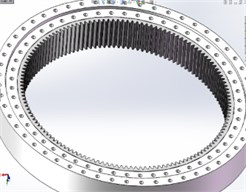a) Geometric model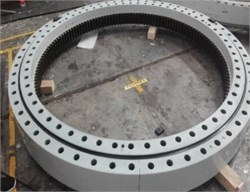b) Physical model

Fig. 2Pitch bearing (with weak zone)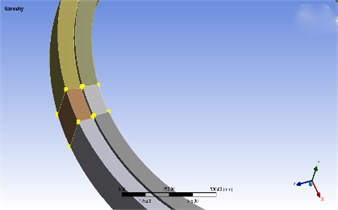Fig. 3Detail of meshing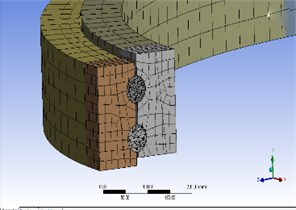### 3.2. Meshing of weak area

This paper selects the automatic division method. If the geometry cannot be swept, the program automatically generates a tetrahedron, and vice versa produces a hexahedron . Meshing produces a total of 188,054 cells and 355,992 nodes, as shown in Fig. 3.

The pitch bearings are an important part of the wind turbine pitch system. Its outer ring is fixed to the hub, and the inner ring is connected to the blade. According to the actual working condition of the bearing, the outer ring is completely restrained, and the axial load, radial load and overturning moment are applied to the bearing.

The following are the various external loads acting on the pitch bearing under four extreme conditions .

Table 3External load under extreme conditions

 Design load condition (DLC) ${F}_{a}$ (KN) ${F}_{r}$ (KN) $M$ (KN·m) Extreme running gust 3599 725 942.13 Extreme turbulence fatigue model 1764 1418 2155.28 Extreme wind speed model –252 999 1297.97 Extreme wind shear model 2053 1418 2210.64

### 3.4. Contact pair setting

In this paper, a total of 960 contact pairs need to be defined, as shown in Fig. 4. In these contact pairs, the surface of the steel ball serves as a contact surface, and the four raceway surfaces serve as target surfaces.

Fig. 4Contact pair setting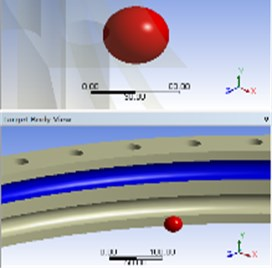### 3.5. Simulation result of finite element

From the Eqs. (1)-(4), the force of the pitch bearing under various working conditions can be obtained, as shown in the Table 4.

The figure below shows the results of finite element calculation.

 Working condition Theoretical value (KN) Theoretical maximum contact load (MPa) Extreme running gust 41.439 1413.26 Extreme turbulence fatigue model 53.843 1836.29 Extreme wind speed model 26.390 900.02 Extreme wind shear model 56.233 1917.80

Fig. 5Load on pitch bearing in the first condition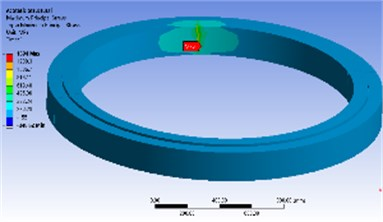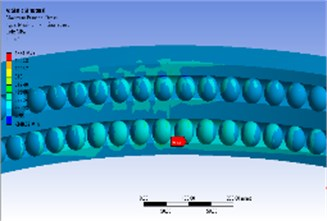Fig. 6Load on pitch bearing in the second condition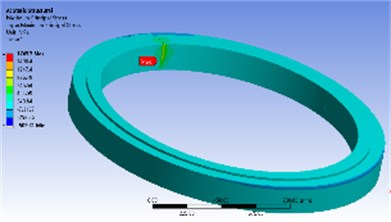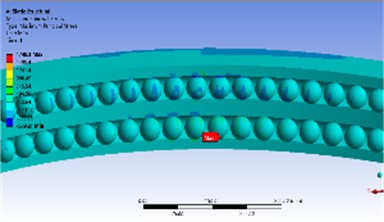Fig. 7Load on pitch bearing in the third condition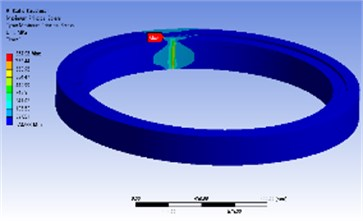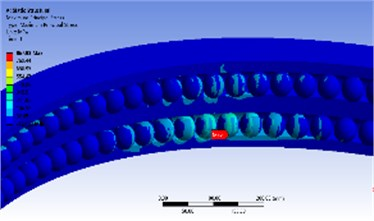Fig. 8Load on pitch bearing in the fourth condition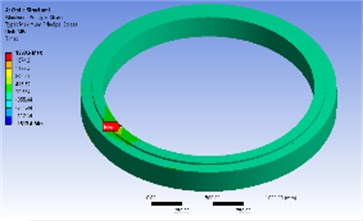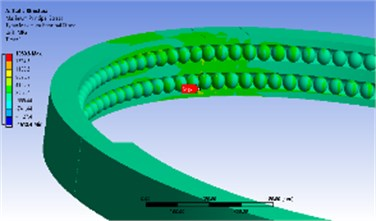## 4. Result analysis

Table 5 shows the deviation of the finite element solution and the theoretical solution. It is easy to see that the error between the finite element value and the theoretical value is less than 5 %.

Table 5Deviation between theoretical value and simulated value

 Working condition Theoretical maximum contact load (MPa) Finite element maximum contact load (MPa) Deviation (%) Extreme running gust 1413.26 1394.0 1.4 Extreme turbulence fatigue model 1836.29 1749.3 4.7 Extreme wind speed model 900.02 867.92 3.5 Extreme wind shear model 1917.80 1960.6 2.2

## 5. Conclusions

1) Results of the finite element and the theoretical value of the empirical formula have a good consistency.

2) According to the comparative analysis of Figs. 5-8, the contact stress at the weak zone of the pitch bearing is larger than the contact stress of the quenching zone. The maximum contact stress is at the contact point between the bottom end and the inner and outer raceways. Moreover, under different working conditions, the load distribution law of the bearing weak zone is also different. Therefore, in the actual installation process, the bearing weak zone should be kept away from the force zone.

3) The simulation analysis of the bearing’s weak zone is a very complicated project. This paper only performs a simple load analysis on the weak zone of the pitch bearing. In the next studies, the bearing capacity and fracture mechanism of pitch bearings with weak zones became an urgent problem to be solved.

#### Cited by

Wear in wind turbine pitch bearings—A comparative design study

31 March 2019
Accepted
21 April 2019
Published
25 June 2019
SUBJECTS
Mechanical vibrations and applications
Keywords
pitch bearing
non-quenching zone
static analysis